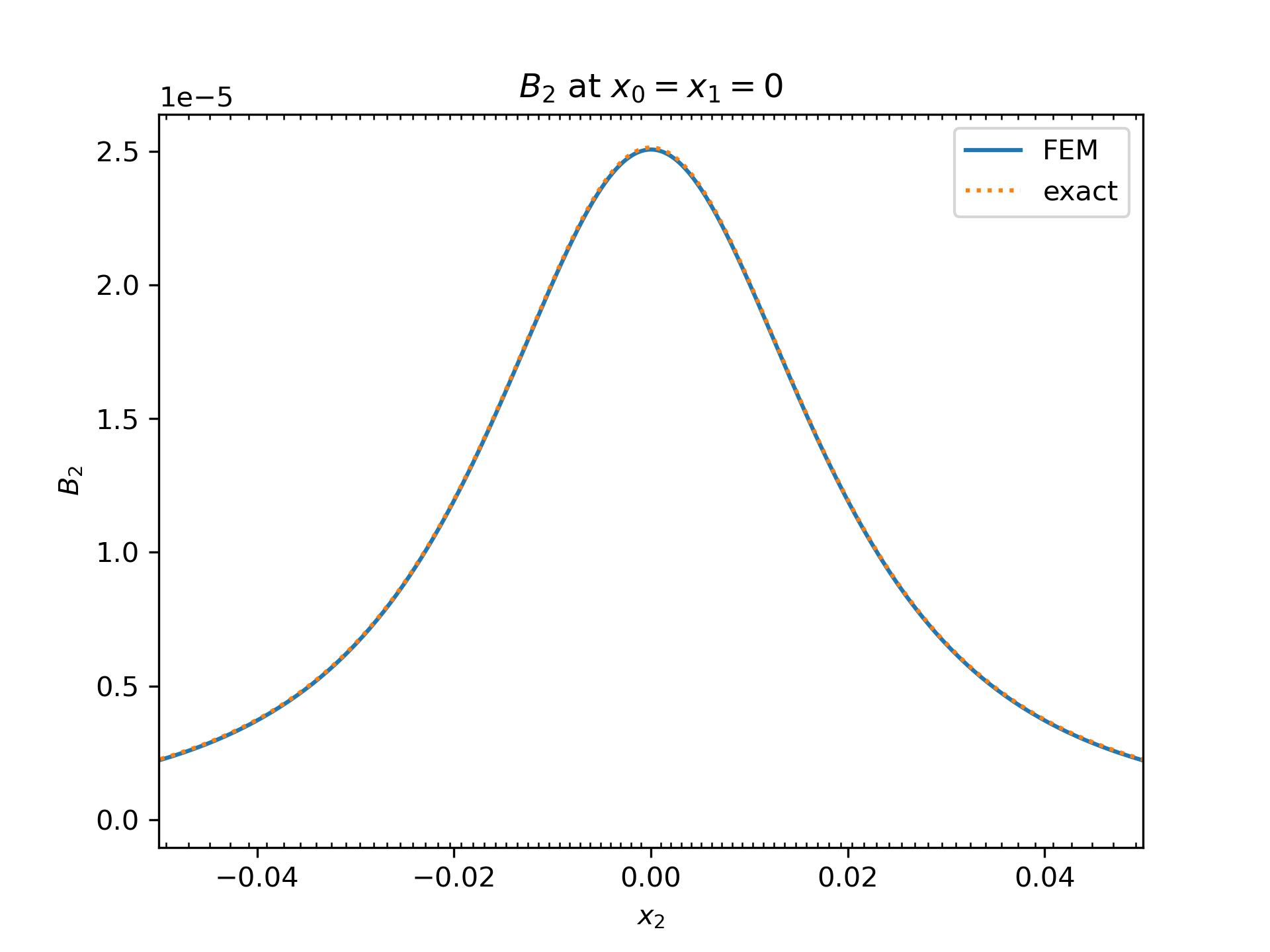By Evalf, and other Nutils contributors

officialelectro-magnetism

Computes the magnetic field induced by a DC or AC current in one or several toroidal conductors. This problem is modeled with the quasi-static magnetic vector potential with Lorenz gauge:

∇_j(∇_j(A_i)) = -μ0 J_i


where A is the magnetic vector potential, J the current density and μ0 the magnetic permeability. The magnetic field B is then given by the curl of the magnetic vector potential. The current density is the sum of an external current Jext and the current induced by the magnetic field, Jind. The external current is given by

Jext_i = (I / π rwire²) cos(ω t) eθ_i


inside the conductor and zero everywhere else, where ω = 2 π f. The induced current follows from Faraday's law of induction and Ohm's law:

Jind_i = -σ ∂_t(A_i)


where σ is the conductivity, which is non-zero only inside the conductor.

We can solve the temporal component of A by letting A_i = Re(Â_i exp(j ω t)). This problem in terms of Â is:

∇_j(∇_j(Â_i) = -μ0 Ĵ_i


with

Ĵext_i = (I / π rwire²) eθ_i


and

Ĵind_i = -j ω σ Â_i